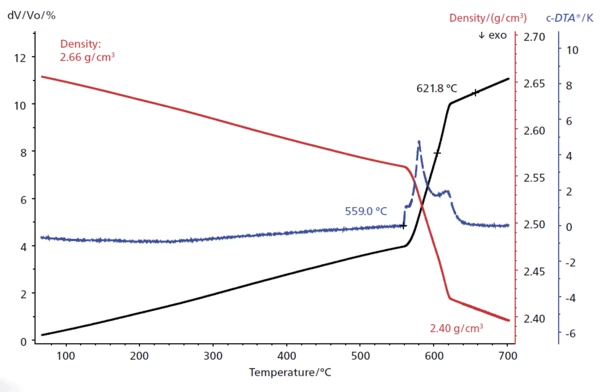Glossary

# Volumetric Expansion

The volume of a gas, solid or liquid changes if the temperature, the pressure or the forces acting on that gas/solid/liquid change. In the case of thermal analysis, we are looking at temperature-dependent changes.

The volume expansion can be measured directly by means of what is known as a volume dilatometer, or indirectly for isotropic materials by measuring the linear thermal expansion and then calculating the volume expansion using special software. The formula for temperature-dependent volume expansion is:

ΔV = Υ⋅V0⋅ΔT

ΔV = volume change in m3
Y = volumetric thermal expansion coefficient in 1/K
V0 = initial volume in m3
ΔT = temperature change in K

Anisotropic materials (e.g., fiber-reinforced polymers) exhibit different thermal expansion depending on the direction of the measurement. For these materials, calculation of the volume expansion is more complex.

See an example below where the volume expansion (dV/V0) was calculated based on the measured thermal expansion using special software. The curve for the volume expansion shows a steep increase in the temperature range from 560°C to 620°C due to the melting of the sample; the DensityThe mass density is defined as the ratio between mass and volume. density decreases significantly in the same range.Thermal behavior of an aluminum-based alloy, heating rate: 5 K/min, He atmosphere, constant contact force: 250 mN, alumina sample holder, alumina container. Displayed are the volumetric expansion (black solid line), the curve of the calculated DensityThe mass density is defined as the ratio between mass and volume. density change (red solid line) and the c-DTA®® curve (blue, dashed line).